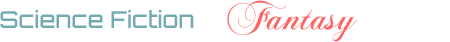## Avid Users Rep Score Targets

3

(click on this box to dismiss)Q&A for science fiction and fantasy enthusiasts

``````declare @target_20k float
declare @target_10k float
declare @target_3k float
declare @target_2k float

set @target_20k = 3.0
set @target_10k = 10.0
set @target_3k = 80.0
set @target_2k = 120.0

/* only look at users that visited the site recently */
declare @lastActiveDays int
set @lastActiveDays = 60

select cast([UserCount20K] as varchar(6)) + '/' + cast(@target_20k as varchar(6)) + ' = ' + cast([20K] as varchar(6)) + '%' [20K],
cast([UserCount10K] as varchar(6)) + '/' + cast(@target_10k as varchar(6)) + ' = ' + cast([10K] as varchar(6)) + '%' [10K],
cast([UserCount3K]  as varchar(6)) + '/' + cast(@target_3k  as varchar(6)) + ' = ' + cast([3K] as varchar(6)) + '%' [3K],
cast([UserCount2K]  as varchar(6)) + '/' + cast(@target_2k  as varchar(6)) + ' = ' + cast([2K] as varchar(6)) + '%' [2K],
[TotalSiteRep],
[UsersCount],
round([TotalSiteRep]/cast([UsersCount] as float),2) [AvgRep],
[AvidUsersRep],
cast(round([AvidUsersRep]/cast([TotalSiteRep] as float)*100,2) as varchar(6)) + '%' [%AvidRep],
[AvidUsersCount],
cast(round([AvidUsersCount]/cast([UsersCount] as float)*100,2) as varchar(6)) + '%' [%AvidUsers],
round([AvidUsersRep]/cast(AvidUsersCount as float),2) [AvgAvidRep]
from (

select sum([20K]) [UserCount20K],
sum([10K]) [UserCount10K],
sum([3K]) [UserCount3K],
sum([2K]) [UserCount2K],
round(sum([20K])/@target_20k*100,2) [20K],
round(sum([10K])/@target_10k*100,2) [10K],
round(sum([3K])/@target_3k*100,2) [3K],
round(sum([2K])/@target_2k*100,2) [2K],
sum(Reputation) [TotalSiteRep],
count(*) [UsersCount],
sum(AvidRep) [AvidUsersRep],
sum(AvidUser) [AvidUsersCount]
from (
select Reputation,
case when Reputation >= 20000 then 1 else 0 end [20K],
case when Reputation >= 10000 then 1 else 0 end [10K],
case when Reputation >= 3000 then 1 else 0 end [3K],
case when Reputation >= 2000 then 1 else 0 end [2K],
case when Reputation >= 150 then Reputation else 0 end [AvidRep],
case when Reputation >= 150 then 1 else 0 end [AvidUser]
from Users
where datediff(d, LastAccessDate, getdate()) <= @lastActiveDays
) q
) r``````

### Enter Parameters

Options:
-Hold tight while we fetch your results
:records returned in :time ms:cached